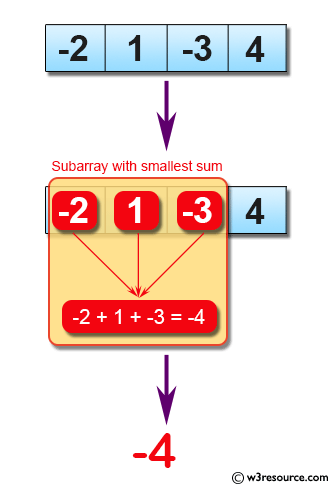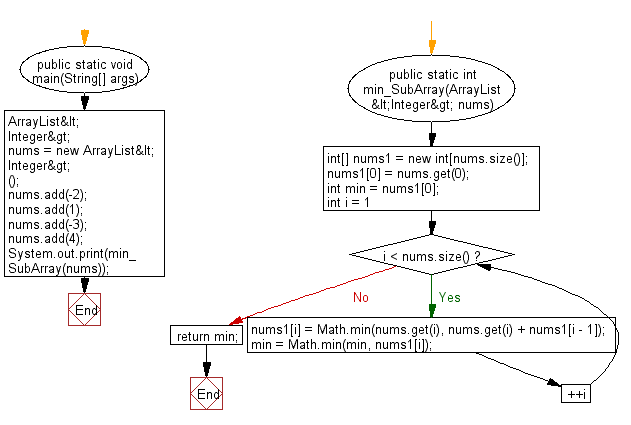﻿ Java: Find the subarray with smallest sum from an array# Java Exercises: Find the subarray with smallest sum from a given array of integers.

## Java Basic: Exercise-123 with Solution

Write a Java program to find the subarray with smallest sum from a given array of integers.

Pictorial Presentation:Sample Solution:

Java Code:

``````import java.util.*;
public class Main {
public static void main(String[] args) {
ArrayList<Integer> nums = new ArrayList<Integer>();
System.out.print(min_SubArray(nums));
}
public static int min_SubArray(ArrayList<Integer> nums) {
int[] nums1 = new int[nums.size()];
nums1 = nums.get(0);
int min = nums1;
for (int i = 1; i < nums.size(); ++i) {
nums1[i] = Math.min(nums.get(i), nums.get(i) + nums1[i - 1]);
min = Math.min(min, nums1[i]);
}
return min;
}
}
```
```

Sample Output:

```-4
```

Flowchart:Java Code Editor:

What is the difficulty level of this exercise?

Test your Programming skills with w3resource's quiz.

﻿

## Java: Tips of the Day

countOccurrences

Counts the occurrences of a value in an array.

Use Arrays.stream().filter().count() to count total number of values that equals the specified value.

```public static long countOccurrences(int[] numbers, int value) {
return Arrays.stream(numbers)
.filter(number -> number == value)
.count();
}
```

Ref: https://bit.ly/3kCAgLb